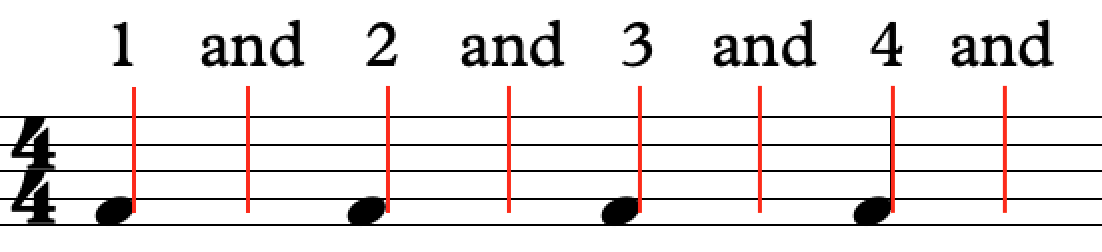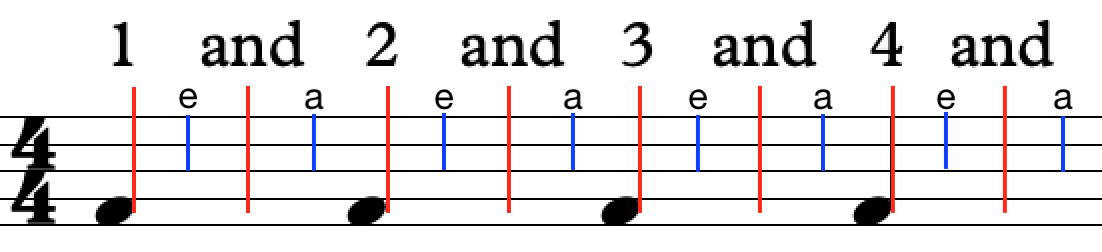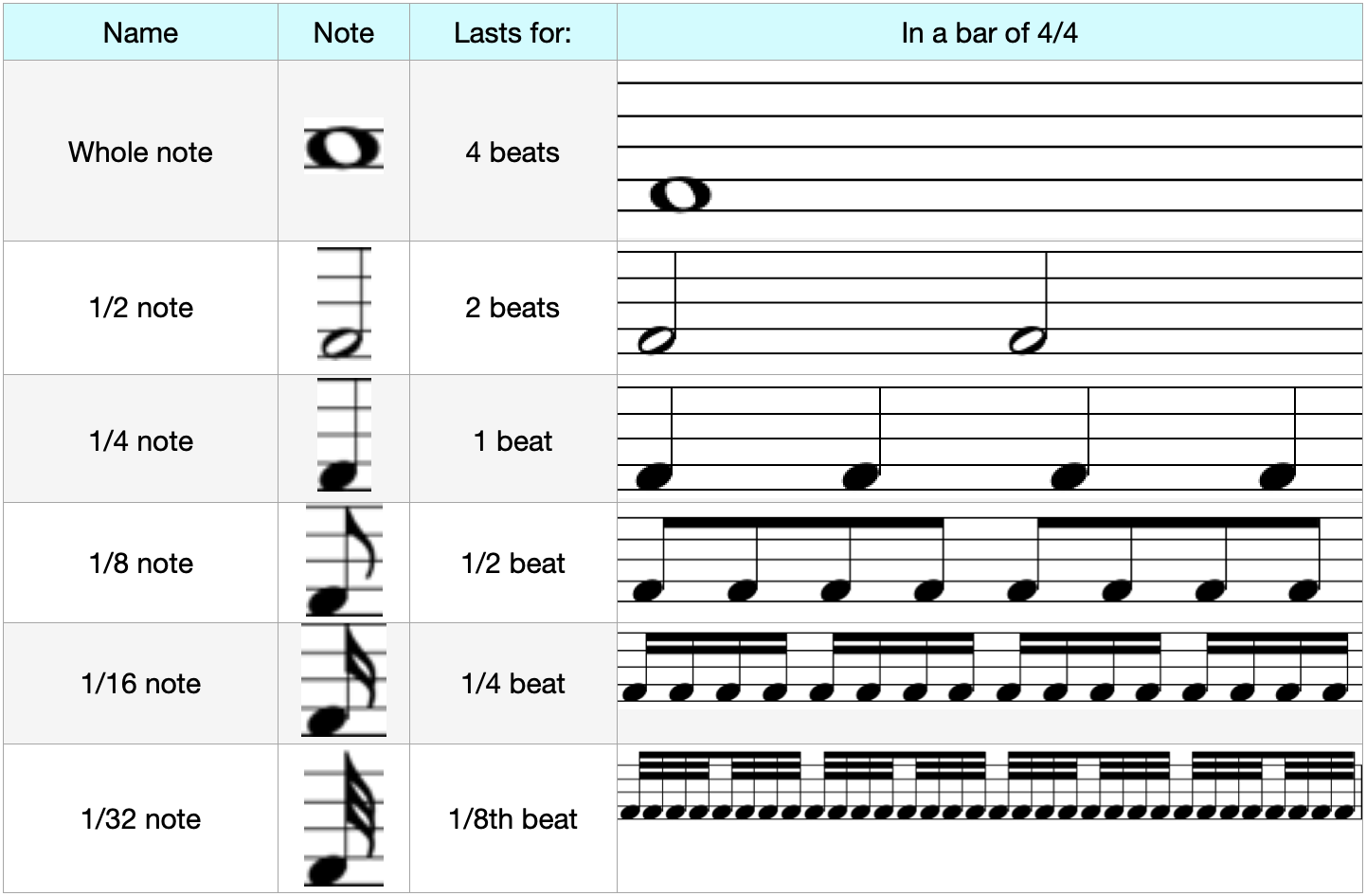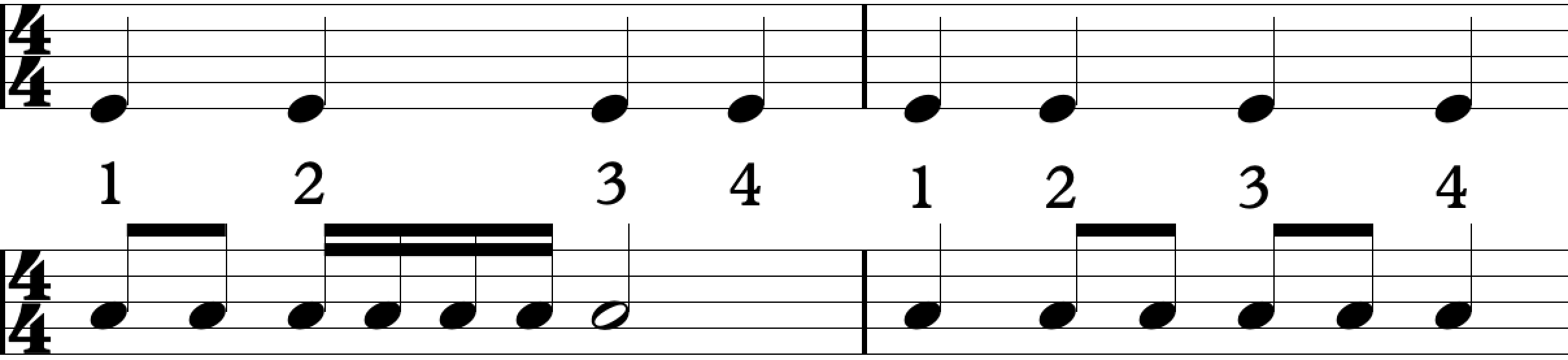# Rhythm Explained: Part 2

So we know what a beat and what rhythm is. Now there is a little more that we need to know that will make learning songs a lot easier!

We're going to look at what note lengths are why why they are useful to us. Last lesson we broke down beats in to:

1, and, 2, and, 3, and, 4, and

2, and, 2, and, 3, and, 4, and

3, and, 2, and, 3, and, 4, and

4, and, 2, and, 3, and, 4, and

This is what it looks like on a stave. The black dots are the beat and the red lines are the "and"s.Now we can break this down even further. We can have "1 e and a" so we break it up in to 4 sub-beats for every beat. So we would count it like this:

1 e and a 2 e and a 3 e and a 4 e and a

2 e and a 2 e and a 3 e and a 4 e and a

3 e and a 2 e and a 3 e and a 4 e and a

4 e and a 2 e and a 3 e and a 4 e and aWe can fit a certain number of notes in to this space. We can choose which notes to put in from the list below, and we can create thousands of melodies with our options.

We can have any combination of these notes to create different melodies. In short, the lengths half every time we go down. The fast notes have a 'tail' on the top of them and the slower notes generally have no fill in the dot.Okay, cool. So we know how long notes last, and we can have as many rhythms as we like, but we will need to put them in sections otherwise we might get lost with where we are.

We have a thing in music called a time signature. This tells us how much time we have in a bar. This is how they look:So the 2 numbers tell us different things. The bottom one tells us what type of note we have to use. in a 4/4 time signature we use a 1/4 note as our reference. We use a 4 because it is the name of that note. The bottom number will usually only be 2, 4, 8, 16.

The top number tells us how many 1/4 notes we have to use. With 4/4, we can put any rhythm that matches the value of 4x 1/4 notes. Have a look below and see how they are the same.If we had a 6/8 time signature, we would use 6x 1/8th notes. They have a different feel to them. Listen to the examples below and you will hear what I mean!

We're learning this because it will make it easier for you to visualise rhythms, you will start to understand them better and learn songs quicker.

In summary, a Time Signature tells us how many beats there are in a bar. So if a time signature is 4/4, that means there are 4 lots of quarter note (1/4) beats. If it were 5/8 there would be 5 lots of 8th notes (1/8).